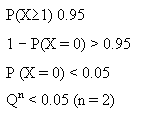Courses

# Test: Engineering Mathematics & General Aptitude - 2

## 20 Questions MCQ Test GATE ECE (Electronics) 2022 Mock Test Series | Test: Engineering Mathematics & General Aptitude - 2

Description
This mock test of Test: Engineering Mathematics & General Aptitude - 2 for Electronics and Communication Engineering (ECE) helps you for every Electronics and Communication Engineering (ECE) entrance exam. This contains 20 Multiple Choice Questions for Electronics and Communication Engineering (ECE) Test: Engineering Mathematics & General Aptitude - 2 (mcq) to study with solutions a complete question bank. The solved questions answers in this Test: Engineering Mathematics & General Aptitude - 2 quiz give you a good mix of easy questions and tough questions. Electronics and Communication Engineering (ECE) students definitely take this Test: Engineering Mathematics & General Aptitude - 2 exercise for a better result in the exam. You can find other Test: Engineering Mathematics & General Aptitude - 2 extra questions, long questions & short questions for Electronics and Communication Engineering (ECE) on EduRev as well by searching above.
QUESTION: 1

### Two pipes A and B can fill a cistern in 37*1/2 minutes and 45 minutes respectively. Both pipes are opened. The cistern will be filled in just half an hour, if' the B is turned off after _______ min

Solution:

Let B be turned off after x minutes. Then
Part filled by (A+B) in x mm. + part filled by A in (30 -x) mm. = 1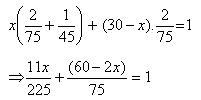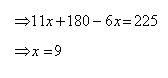QUESTION: 2

### A boat can travel with a speed of 13 km/hr in still water. If the speed of the stream is 4 km/hr, find the time taken by the boat to go 68 km downstream.

Solution:

A boat can travel with a speed of 13 km/hr in still water. If the speed of the stream is 4 km/hr, find the time taken by the boat to go 68 km downstream.
Speed down stream = (13+4) km/hr = 17 km/hr.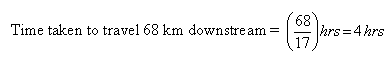QUESTION: 3

### What is the probability of getting a sum 9 from two throws of a dice?

Solution: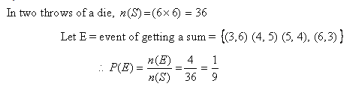QUESTION: 4

A Child is looking for his father. He went 90 meters in the east before turning to his right. We went 20 meters before turning to his right again to look for his father at his uncle’s place 30 meters from this point. His father was not there. From there, he went 100 meters to his north before meeting his father in a street. How far did the son meet his father from starting point?

Solution:

Clearly, the child moves from A 90 m east words upto B, then turns right and moves 20 m upto C, then turns right and moves 30 m upto D Finally he turns right and moves 100 m upto E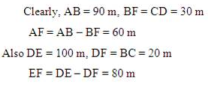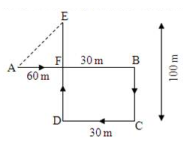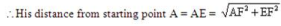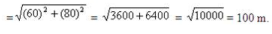QUESTION: 5

A and B are brothers C and D are sisters. A’s son is D’s brother. How is B related to C?

Solution:

The above problem can be solved from following blood relation tree.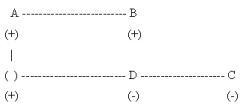Clearly, A is the C’s father. So, the Father’s Brother is uncle, Hence, the answer (D).

QUESTION: 6

Choose the box that is similar to the box formed from the given sheet of paper (X).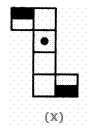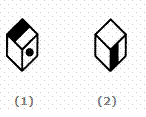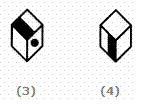Solution:

The fig. (X) is similar to Form II. So, when a cube is formed by folding the sheet shown in fig. (X), then the two half-shaded faces lie opposite to each other and one. of the three blank faces appears opposite to the face bearing a dot. Clearly, each one of the four cubes shown in figures (1), (2), (3) and (4) can be formed by folding the sheet shown in fig. (X).

QUESTION: 7

Select a suitable figure from the Answer Figures that would replace the question mark (?).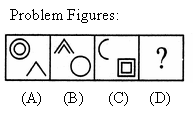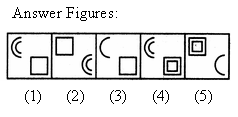Solution:

Each one of the upper elements is replaced by an element similar to the lower elernent(s) and each one of the lower elements is replaced by an element similar to the upper element(s).

QUESTION: 8

The bar graph given below shows the sales of books (in thousand number) from six branches of a publishing company during two consecutive years 2000 and 2001.
Sales of Books (in thousand numbers) from Six Branches - Bl, B2, B3, B4, B5 and B6 of a publishing Company in 2000 and 2001.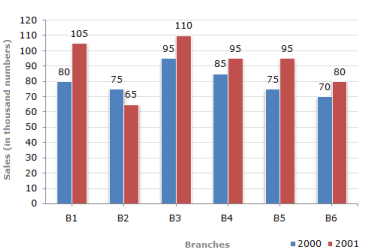Q.

What is the ratio of the total sales of branch B2 for both years to the total sales of branch B4 for both years?

Solution:
QUESTION: 9

The bar graph given below shows the sales of books (in thousand number) from six branches of a publishing company during two consecutive years 2000 and 2001.
Sales of Books (in thousand numbers) from Six Branches - Bl, B2, B3, B4, B5 and B6 of a publishing Company in 2000 and 2001.Q.

Total sales of branch B6 for both the years is what percent of the total sales of branches B3 for both the years

Solution: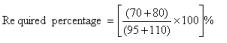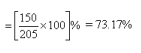QUESTION: 10

The bar graph given below shows the sales of books (in thousand number) from six branches of a publishing company during two consecutive years 2000 and 2001.
Sales of Books (in thousand numbers) from Six Branches - Bl, B2, B3, B4, B5 and B6 of a publishing Company in 2000 and 2001.Q.

What percent of the average sales of branches Bl, B2 and B3 in 2001 is the average sales of branches Bl, B3 and B6 in 2000?

Solution:

Average sales (in thousand number) of branches Bl, B3 and B6 in 2000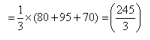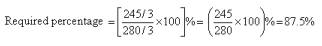QUESTION: 11

The values ofk, for which the equations x + y+ z = 1, x+2y + 4z = k and x + 4y + 10z = k2 have at least one solution are

Solution: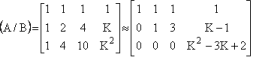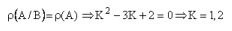QUESTION: 12

All eigen values of the matrix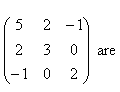Solution:

AT=A
Eigen value of real symmetric matrix are real.

QUESTION: 13

For the equation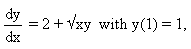then y(1.1) by using Euler’s method is

Solution: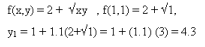QUESTION: 14

The function f(x, y) = x2+ bxy + y2 has relative minimum at

Solution: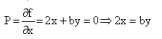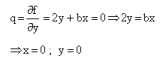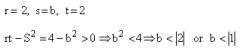QUESTION: 15

If f(x,y) = y, g(x,y) = x2 (L is the line segment from (1,1) to (3,5)) then the line integral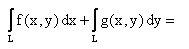Solution:
QUESTION: 16

An article manufactured by a company consists of two parts x & y. In the process of manufacturing the parts x, 9 out of 104 parts may be defective. Similarly 5 out of 100 are likely to be defective in manufacturing the part y. The probability that the assembled product will not be defective is

Solution: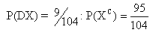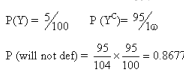QUESTION: 17

If U(x, y)=x2-y2 -3x in an analytic function f(z)=U(x, y)+ lV(x, y) then V(x, y) is

Solution:
QUESTION: 18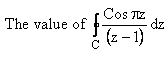taken along the curve C: | z - 1 | = 2 in the positive direction is

Solution: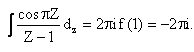QUESTION: 19

There are ‘n’ persons sitting in a row, two of them are selected at random the probability that two selected persons are not together is

Solution: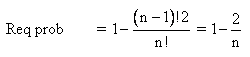QUESTION: 20

The minimum of dice are thrown for getting sums at least once greater than 0.95 is ________

Solution: